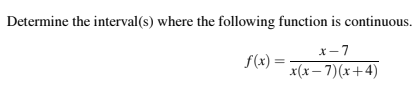Determine the interval (s) where the following function is continuousx-7f(x)= (x-7)x+4)

Questionhelp_outlineImage TranscriptioncloseDetermine the interval (s) where the following function is continuous x-7 f(x)= (x-7)x+4) fullscreen
Step 1

In the given function (x-7) is a common factor in numerator and denominator. So, it will cancel out and it gives the more simplified function.

Step 2

A rational function is continuous for all real numbers except at points where its denominator is 0.
So, in order to find the interval of continuity for the function we have to find a interval of x for which the denominato...

Want to see the full answer?

See Solution

Want to see this answer and more?

Our solutions are written by experts, many with advanced degrees, and available 24/7

See Solution
Tagged in

Continuity